S
15 posts

Implementing a Font Reader and Rasterizer from Scratch, Part 1: TTF Font Reader.
8 months, 2 weeks ago Edited by S on May 14, 2020, 7:23 p.m. Reason: First link was broken.

I had been wanting to write this for over a year, yes you read that right a year, I will explain why at the end as its an optional read.

Notes:
1. The main reference I'm using is (Apple TrueType Reference Manual),
I have done my best to write it in way that made sense to me and how I understood it, if you find any mistakes please do let me know.
2. all the code snippets I'm using in different sections are going to be collected in one final single file that can be compiled and ran.
3. I will use the font Envy Code R (Envy Code R) for this post.
4. Most of the code here is for education and might contain oversight when it comes to memory management, type sizes, error checking etc.

I will write this in two parts first reading the TTF file then rasterizing the glyph outlines, a nice break point between the two processes.
Lets start

Part 1: TTFs

1. Terminology:

I'm not a font designer nor even remotely someone who knows anything too detail about them but there are some terminology we should know before going forward:

• Typeface: this is basically the design of the font and what it actually looks like for example Arial is a typeface
Arial Regular is a variant of the Arial typeface which you call a font, Arial Bold is another font.
• Font: a variant of a typeface such as a bold or italic variant of a specific typeface. a Font is made up of collection of glyphs.
• Character: this is a specific symbol that represents a letter, number or some other sign (such as dollar sign \$) in written language.
• Glyph: a shape/symbol that represents a character in writing having a particular form. for example the glyph for the letter A in the Envy Code R typeface looks like this: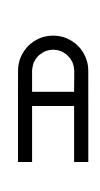• Codepoint: an integer that represents a Character index in an encoding scheme, the two most common encoding schemes are (ASCII and Unicode) ASCII uses one byte for the Codepoint hence it only has 255 Codepoints, unicode uses multiple bytes for its Codepoints thus it can encode a much larger range.
• Glyph Index: this is an interger that gives you the location of a Glyph inside a font, this changes depending on the font unlike Codepoints which are specific to the encoding scheme (Unicode or ASCII) rather than the font.

Our main point of knowing these terms is that we distinguish a Glyph from a Character in that a Glyph is a representation of a Character in a particular Typeface as in the uppercase A character doesn't change as there is one uppercase A letter in the english language while a Glyph for the uppercase A are plenty and depends on the Typeface

2. Intro

Truetype Fonts are files that contain information regarding a particular typeface, they contain each of the typeface glyph's outlines, these outlines are bezier curves.The file also includes specific information about grid-fitting a glyph also known as (hinting),
its positioning and a host of other information about the typeface.
Our main business will be reading the outline data and rasterize, this means this post won't include hinting and other font stuff like shaping and substitutions that is needed for non-English languages.

I will do a separate post on substitution and simple shaping at a later date.

TTFs files use a format that is called SFNT (Spline Font or Scalable Font) which is a file format that houses other fonts such as PostScript fonts, OpenType fonts, TrueType fonts, they have the general overall structure but different in certain parts.
SFNT was superseded by OpenType Collections which is an extended version of SFNT.

TTF files do not contain any complex compression thus making most of our work just byte reading, all the data is in *big endian*.

before we even begin, lets write some small snippets of code to help us make life easier. first we need to read an entire file:

 ``` 1 2 3 4 5 6 7 8 9 10 11 12 13 14 15 16 17 18 19 20 21 22 23``` ```char* read_file(char *file_name, int* file_size) { if(strlen(file_name) > 0) { FILE* file = fopen(file_name, "rb"); if(file) { fseek(file, 0, SEEK_END); int size = ftell(file); fseek(file, 0, SEEK_SET); if(file_size) { *file_size = size; } char *file_content = (char*)malloc(size+1); int read_amount = fread(file_content, size, 1, file); file_content[size] = '\0'; if(read_amount) { fclose(file); return file_content; } free(file_content); fclose(file); return NULL; } } return NULL; } ```

Now we need to read big endian values, we assume mem is char*,it can also be a uint8_t which is one of the standard int sizes but less dependency on libraries the better, we technically only need 16 and 32 bytereading as you don't have bigger than 32 bit sized types that we care about. the only 64 bit type is longdatetime which is the usual unix epoch timestamp.

we are also going to define some data types to make writing unsigned int a bit shorter

 ``` 1 2 3 4 5 6 7 8 9 10 11 12 13 14 15``` ```typedef unsigned char u8; typedef char i8; typedef unsigned short u16; typedef short i16; typedef unsigned int u32; typedef int i32; #define READ_BE16(mem) ((((u8*)(mem)) << 8) | (((u8*)(mem)))) #define READ_BE32(mem) ((((u8*)(mem)) << 24) | (((u8*)(mem)) << 16) | (((u8*)(mem)) << 8) | (((u8*)(mem)))) #define P_MOVE(mem, a) ((mem) += (a)) #define READ_BE16_MOVE(mem) (READ_BE16((mem))); (P_MOVE((mem), 2)) #define READ_BE32_MOVE(mem) (READ_BE32((mem))); (P_MOVE((mem), 4)) ```

if you notice I haven't distinguished between signed and unsigned macros because the reading of the data is the same but the interpretation of the data is dependent on the type read into.

The font files are made up of tables that contain different information. Each table has a 4 byte tag which can be seen as an uint32 or 4 characters, these tags are not stored with the table data rather they are stored in a special table called the table directory which comes at he start of the file and identifies the offsets of all the other tables along with their tag.

The file structure starts with a table called the font directory and it must be the first table in the file, it consists of 2 parts; the offset subtable and table directory, offset subtable contains info on the amount of tables and provides some precalculated numbers to help with binary searching for a particular table, that is if the number of tables is huge or if you just want a faster search than linear,
this binary search is very useful when you want to search for a table and you haven't extracted that tables information into a specific data structure and don't read all the tables in one go.
table directory is an array that contains the tags of the table and their location offsets in bytes,

this is how we will define the struct for the font directory

 ```1 2 3 4``` ```typedef struct { offset_subtable off_sub; table_directory* tbl_dir; } font_directoy; ```

4. Offset Subtable

Here is the structure for the Offset subtable:

 ```1 2 3 4 5 6 7``` ```typedef struct { u32 scaler_type; u16 numTables; u16 searchRange; u16 entrySelector; u16 rangeShift; } offset_subtable; ```

the most important piece of info we need is of course the numTables this will dicatet
how many tables there.
scaler_type
• a value of 0x74727565 ('true' as chars) or 0x00010000 are considered TrueType fonts
• a value of 0x4F54544F ('OTTO' as chars) means its an OpenType with PostScript outlines
• a value of 0x74797031 ('typ1' as chars) is type 1 postscript font files which was made by adobe in the 80s

the focus of this post is about the first values, the other values are different and not as common.
the searchRange, entrySelector and rangeShift are all used for a binary search for a specific tag which we will not indulge here as a simple linear is fine for us.

5. Table Directory

Here is the structure:

 ```1 2 3 4 5 6 7 8 9``` ```typedef struct { union { char tag_c; u32 tag; }; u32 checkSum; u32 offset; u32 length; } table_directory; ```

the offset is taken as an offset from the start of the file and not from table_directory

what follows the Offset table is actually an array of (table_directory) the number of items in the array is given by the field numTables from offset_subtable struct. The union is for easier debugging as the 4 character version is easier to read as humans one small note about the tag if you read them as big endians they come out reversed so a tag of cmap becomes pamc

Now that we have at least two structs, lets read some data, we begin by reading font_directory which is nothing else than reading the offset_subtable and table_directory,

 ```1 2 3 4``` ```void read_font_directory(char** mem, font_directory* ft) { read_offset_subtable(mem, &ft->off_sub); read_table_directory(mem, &ft->tbl_dir, ft->off_sub.numTables); } ```

the reason for the char** is that we are going to use it as a pointer inside the data stream of the file.

 ```1 2 3 4 5 6 7 8 9``` ```void read_offset_subtable(char** mem, offset_subtable* off_sub) { char* m = *mem; off_sub->scaler_type = READ_BE32_MOVE(m); off_sub->numTables = READ_BE16_MOVE(m); off_sub->searchRange = READ_BE16_MOVE(m); off_sub->entrySelector = READ_BE16_MOVE(m); off_sub->rangeShift = READ_BE16_MOVE(m); *mem = m; } ```

et voila we have the offset_table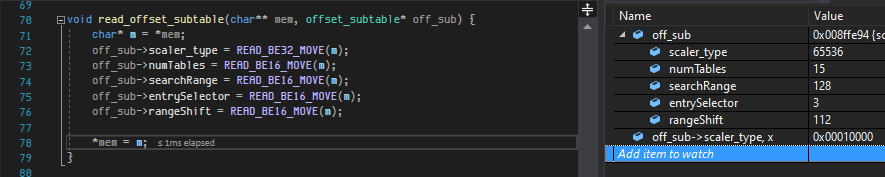very simple and straight to the point, then we read the table_directory:

 ``` 1 2 3 4 5 6 7 8 9 10 11 12 13 14``` ```void read_table_directory(char** mem, table_directory** tbl_dir, int tbl_size) { char* m = *mem; *tbl_dir = (table_directory*)calloc(1, sizeof(table_directory)*tbl_size); for(int i = 0; i < tbl_size; ++i) { table_directory* t = *tbl_dir + i; t->tag = READ_BE32_MOVE(m); t->checkSum = READ_BE32_MOVE(m); t->offset = READ_BE32_MOVE(m); t->length = READ_BE32_MOVE(m); } *mem = m; } ```

we first get the size of the table_directory and the rest is easy reading.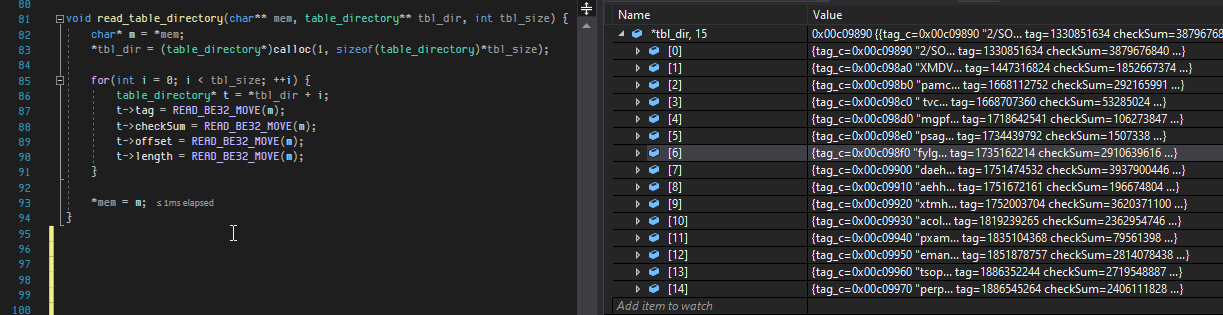to make it easier to see what we have read we are going to write a print function:

 ``` 1 2 3 4 5 6 7 8 9 10``` ```void print_table_directory(table_directory* tbl_dir, int tbl_size) { printf("#)\ttag\tlen\toffset\n"); for(int i = 0; i < tbl_size; ++i) { table_directory* t = tbl_dir + i; printf("%d)\t%c%c%c%c\t%d\t%d\n", i+1, t->tag_c, t->tag_c, t->tag_c, t->tag_c, t->length, t->offset); } } ```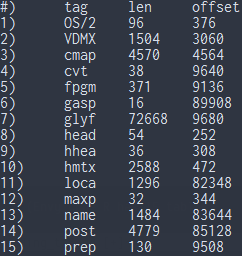(Envy Code R has 15 tables)
now that is much better to read.

6. the tables that we need

Given that we are ready to read some more data we move on onto our main quest,

All TTF files (TrueType variants) are required to have the following tables in them.

• cmap: contains the mapping between a paricular Codepoint to Glyph index, i.e: given a character it tells us which Glyph is used to represent that Character in the Typeface
• glyf: contains the actual Glyph outline data, all these are splines.
• hhea: horizontal header table, contains information about horizontal positioning of a font and its glyphs.
• hmtx: an array that has horizontal metrics for each glyph, specifically advance width and left side bearing.
• loca: glyph location offsets, this table contains the offset of each glyph's data, its used together with the 'glyf' table this table is used to locate the Glyph data inside the glyf table.
• maxp: Maximum Profile, this table contains the extends of the font, i.e: the number of glyphs, biggest number of points in a Glyph, etc. basically it gives you the memory constraints of the font such as you can allocate a buffer to contain the Glyph data and this table tells you how big you should make it so it can contain the biggest Glyph.
• name: this table contains the name of the font and human readable data.
• post: we won't touch this table so we are going to skip it.

as you can see these tables all work together to get you the data, glyf table contains the actual data, loca contains the offsets into the glyf table which contain the Glyph outline data, cmap gives you the Codepoint to Glyph mapping so you can get a Glyph Index to use with the loca table, head, hhea, hmtx together give you information about the font layouting and positioning.

we start with cmap one of the more fun tables to work with

7. cmap entered the channel.

this table is a bit complicated if you come across it the first time so I'm gonna try to explain it first then we move on to the structure and reading.

this table is used when you want to map a specific Codepoint to a Glyph index, The cmap table contains a number of subtables which are called encoding subtables because different platforms have developed different conventions when dealing with fonts these encoding subtables help which encoding format should be used on which platform which just means there are different ways the Character to Glyph mapping is encoded and it depends on the platform the font is intended to be used on, a font that is intended for more than one platform would have multiple encoding subtables.

the encoding tables each have a specific format out of nine format that are part of the standard, we will look at one format which is format 4 for now, lets get some structures and begin reading.

cmap begins with a version number and then the number of encoding subtables

 ```1 2 3 4``` ```typedef struct { u16 version; u16 numberSubtables; } cmap; ```

the encoding subtables have the following structure,

 ```1 2 3 4 5``` ```typedef struct { u16 platformID; u16 platformSpecificID; u32 offset; } cmap_encoding_subtable; ```

the offset here is an offset from the start of the cmap table not from the start of the file. Because the encoding subtables follow the cmap we are going to change the cmap struct into

 ```1 2 3 4 5``` ```typedef struct { u16 version; u16 numberSubtables; cmap_encoding_subtable* subtables; } cmap; ```

the platformID and platformSpecificID tell you which platform this encoding subtable
was intended for.

platformID can have these values:
• 0 is called Unicode Platform and is used when the platfrom supports unicode
• 1 is to indicate Mac
• 2 is reserved and not used
• 3 is Microsoft encoding

both TrueType and OpenType specs recommend using the Unicode Platfrom as its more generally supported.
most fonts will provide the Unicode Platform hence that would be our main focus.

when the platformID is 0 the platfromSpecificID can have the following values:

• 0, 1, 2 - these indicate Unicode standard version 1 and 1.1 respectively and a value of 2 is deprecated.
• 3 - Unicode 2.0 with Basic Multilingual Plane only (BMP this is called Plane 0 which contains the languages and a lot of symbols),
• 4 - Unicode 2.0 with non-BMP allowed
• 5 - Unicode Variation Sequences, these represent variation of a Glyph as an example (from unicode.com FAQ) ≠ which is a variation of =.
• 6 - very similar to (4) but can use more formats for the cmap than number 4

all we need to take away from all of this (which is still confusing) is that the most common platformID and platformSpecificID combination is (0, 3), so we are going to focus on that combination only, supporting the other combinations is just a practice in being more complete and doesn't contribute too much to the overall approach

all the encoding subtables point to a cmap which has a specific format. all the formats have some common parts, they all start with a u16 format field, followed by the length of this cmap table then the language code which is only used on Macintosh platformID which in return is discouraged to be used.

so the start of each format is this:

 ```1 2 3 4 5``` ```typedef struct { u16 format; u16 length; u16 language; }; ```

I have purposely left out the name for this struct because its not a complete as each format has a different structure.

Because there are nine formats available we will focus on what our font (Envy Code R) contains when it comes to encoding subtables

here is the function to read cmap:

 ``` 1 2 3 4 5 6 7 8 9 10 11 12 13 14``` ```void read_cmap(char* mem, cmap* c) { char *m = mem; c->version = READ_BE16_MOVE(m); c->numberSubtables = READ_BE16_MOVE(m); c->subtables = (cmap_encoding_subtable*) calloc(1, sizeof(cmap_encoding_subtable)*c->numberSubtables); for(int i = 0; i < c->numberSubtables; ++i) { cmap_encoding_subtable* est = c->subtables + i; est->platformID = READ_BE16_MOVE(m); est->platformSpecificID = READ_BE16_MOVE(m); est->offset = READ_BE32_MOVE(m); } } ```

and one to print it

 ``` 1 2 3 4 5 6 7 8 9 10 11 12 13 14``` ```void print_cmap(cmap* c) { printf("#)\tpId\tpsID\toffset\ttype\n"); for(int i = 0; i < c->numberSubtables; ++i) { cmap_encoding_subtable* cet = c->subtables + i; printf("%d)\t%d\t%d\t%d\t", i+1, cet->platformID, cet->platformSpecificID, cet->offset); switch(cet->platformID) { case 0: printf("Unicode"); break; case 1: printf("Mac"); break; case 2: printf("Not Supported"); break; case 3: printf("Microsoft"); break; } printf("\n"); } } ```

to begin our reading we first need to find the cmap offset in the table_directory using a linear search, here is the code for that, done directly in main because why not:

 ``` 1 2 3 4 5 6 7 8 9 10 11 12 13 14 15 16 17``` ```int main(int argc, char** argv) { int file_size = 0; char* file = read_file("envy.ttf", &file_size); char* mem_ptr = file; font_directory ft = {0}; read_font_directory(&mem_ptr, &ft); for(int i = 0; i < ft.off_sub.numTables; ++i){ if(ft.tbl_dir[i].tag == READ_BE32("cmap")) { cmap c = {0}; char* tbl_ptr = file + ft.tbl_dir[i].offset; read_cmap(tbl_ptr, &c); print_cmap(&c); } } return 0; } ```

After we find the offset from the table_directory we add it to the start of the file and that is whee our cmap begins and we just pass this pointer to read_cmap

here is our result after we read and print the cmap: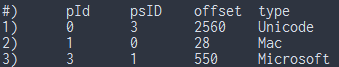We are going to focus on what format the Unicode Platform has

so lets check it: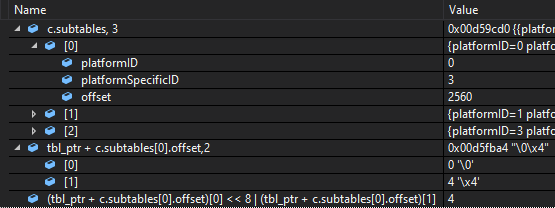as you can see the format value is format 4

8. cmap format 4

format4 is one of the most common formats,
as we know so far cmaps are used to map Codepoints to Glyph indices this mapping of Codepoint to Glyph index is different depending on the format used

format 4 is used when you support the Unicode BMP but not all the Codepoints in the entire plane range (0x0000 to 0xFFFF) thus there are holes in range. this is also indicated by the platformSpecificID (id number 3) for the Unicode Platform.

Note:
the unicode standard is divided into 17 planes which are ranges of Codepoints for letters, symbols, etc of all the different languages, each plane has a size of 16 bits which means each plane contains 2^16 (65,536) Codepoints, the first plane (Plane 0)
is the plane that contains the Codepoints of the languages and a lot of symbols most commonly used and its range is 0x0000 -> 0xFFFF

format 4's structure is very straight forward:

 ``` 1 2 3 4 5 6 7 8 9 10 11 12 13 14 15``` ```typedef struct { u16 format; u16 length; u16 language; u16 segCountX2; u16 searchRange; u16 entrySelector; u16 rangeShift; u16 reservedPad; u16 *endCode; u16 *startCode; u16 *idDelta; u16 *idRangeOffset; u16 *glyphIdArray; } format4; ```

the first 3 fields are the ones we mentioned before that are common across all the formats the searchRange, entrySelector, rangeShift are again used for a binary search (which we will also ignore and use a linear search) the reservedPad field must be zero per the specs.

the startCode, endCode, idDelta, idRangeOffset are 4 parallel arrays that are to be used together. they all have size of segCountX2 divided by 2. the glyphIdArray extends until the end of the table, we will calculate the size from the length field and how many bytes of that length remains after we read the 4 arrays

now then lets read our format 4

 ``` 1 2 3 4 5 6 7 8 9 10 11 12 13 14 15 16 17 18 19 20 21 22 23 24 25 26 27 28 29 30 31 32 33 34 35 36 37 38 39 40 41 42``` ```void read_format4(char* mem, format4** format) { char* m = mem; u16 length = READ_BE16(m + 2); format4* f = NULL; f = (format4*) calloc(1, length + sizeof(u16*)*5); f->format = READ_BE16_MOVE(m); f->length = READ_BE16_MOVE(m); f->language = READ_BE16_MOVE(m); f->segCountX2 = READ_BE16_MOVE(m); f->searchRange = READ_BE16_MOVE(m); f->entrySelector = READ_BE16_MOVE(m); f->rangeShift = READ_BE16_MOVE(m); f->endCode = (u16*) ((u8*)f + sizeof(format4)); f->startCode = f->endCode + f->segCountX2/2; f->idDelta = f->startCode + f->segCountX2/2; f->idRangeOffset = f->idDelta + f->segCountX2/2; f->glyphIdArray = f->idRangeOffset + f->segCountX2/2; char* start_code_start = m + f->segCountX2 + 2; char* id_delta_start = m + f->segCountX2*2 + 2; char* id_range_start = m + f->segCountX2*3 + 2; for(int i = 0; i < f->segCountX2/2; ++i) { f->endCode[i] = READ_BE16(m + i*2); f->startCode[i] = READ_BE16(start_code_start + i*2); f->idDelta[i] = READ_BE16(id_delta_start + i*2); f->idRangeOffset[i] = READ_BE16(id_range_start + i*2); } P_MOVE(m, f->segCountX2*4 + 2); int remaining_bytes = f->length - (m - mem); f->glyph_id_count = remaining_bytes/2; for(int i = 0; i < remaining_bytes/2; ++i) { f->glyphIdArray[i] = READ_BE16_MOVE(m); } *format = f; } ```

The code here is straight forward reading, we get the length first then allocate enough memory for the entire table taking into account memory needed for the pointers of the 5 arrays our format4 struct has, we need to do this because the data of the font doesn't take into account those pointers

We will read the 5 arrays into the end of the memory we allocated, we put the endCode array right after where the format4 struct is in the memory ends, then comes startCode and then the other three arrays after that.

here is a picture to further clarify how the memory allocated is used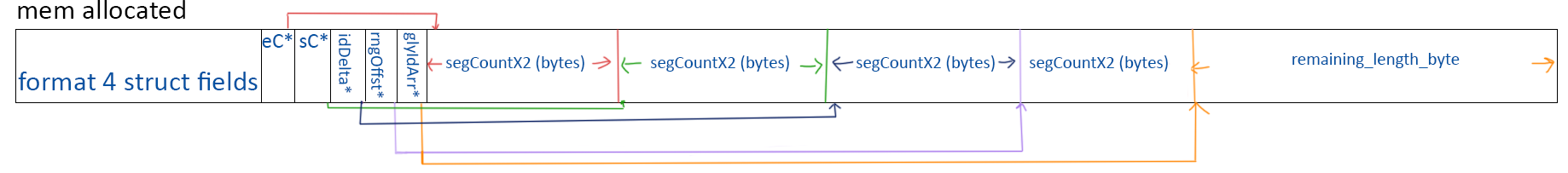my drawing skills leave much to be desired.

lets write a function to print the format4 too.

 ```1 2 3 4 5 6 7 8 9``` ```void print_format4(format4 *f4) { printf("Format: %d, Length: %d, Language: %d, Segment Count: %d\n", f4->format, f4->length, f4->language, f4->segCountX2/2); printf("Search Params: (searchRange: %d, entrySelector: %d, rangeShift: %d)\n", f4->searchRange, f4->entrySelector, f4->rangeShift); printf("Segment Ranges:\tstartCode\tendCode\tidDelta\tidRangeOffset\n"); for(int i = 0; i < f4->segCountX2/2; ++i) { printf("--------------:\t% 9d\t% 7d\t% 7d\t% 12d\n", f4->startCode[i], f4->endCode[i], f4->idDelta[i], f4->idRangeOffset[i]); } } ```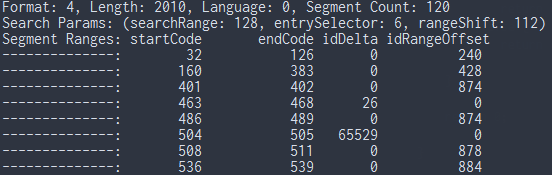the picture doesn't show the entire printed output as it was too big

Now that we have read the cmap table we will begin our mapping by using the startCode, endCode, idRangeOffset, idDelta arrays which at the end will give us a pointer into the glyphIdArray which gives us the final Glyph Index.

9. Fantastic Glyphs and where to find them. (we are looking for glyph indices but that doesn't sound as nice)

Here is how we are going to do mapping of a Codepoint:
1. find the index of the first endCode which is bigger than or equal to the Codepoint. all the arrays are sorted by increasing endCode values.
2. check the corresponding startCode, if its smaller than or equal to the Codepoint then we move to step 3 else we return 0 which means glyph not found.
3. check the corresponding idRangeOffset if its not 0 then go to step 4 otherwise go to step 7
4. take the value from step 3 and add it to the address of the value.
 `1` ```(idRangeOffset[i] + (&idRangeOffset\[i]) ```
5. take the difference between the Codepoint and the startCode and add it to step 4. (
 `1` ```code_point - startCode[i] ```
)
6. the value from the dereferncing the pointer in step 5 is checked, if its 0 then the glyph index is 0 and the glyph is not available. if its not zero we add it to the corresponding idDelta and get the glyph index. skip step 7.
7. if idRangeOffset is 0 then add the Codepoint to the corresponding idDelta to get the glyph index.

seems a bit confusing but once we write the code for this its a bit more clear

 ``` 1 2 3 4 5 6 7 8 9 10 11 12 13 14 15 16 17 18 19 20 21 22``` ```int get_glyph_index(u16 code_point, format4 *f) { int index = -1; u16 *ptr = NULL; for(int i = 0; i < f->segCountX2/2; i++) { if(f->endCode[i] > code_point) {index = i; break;}; } if(index == -1) return 0; if(f->startCode[index] < code_point) { if(f->idRangeOffset[index] != 0) { ptr = f->idRangeOffset + index + f->idRangeOffset[index]/2; ptr += code_point - f->startCode[index]; if(*ptr == 0) return 0; return *ptr + f->idDelta[index]; } else { return code_point + f->idDelta[index]; } } return 0; } ```

all right that is all there is to it for getting Glyph Indexs. you can go ahead and try a couple of different indices.

here is the Glyph Indexs of the uppercase English letters: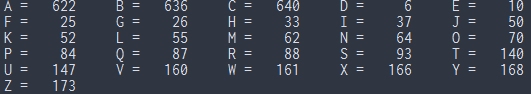10. Break

if you have come this far, take a small break its healthy.

11. 'loca' and the boys

Now that we have the Glyph index mapping working, we move onto getting the Glyph data which is stored in the glyf table, the offset of the Glyph in the glyf is stored in the loca table which is a very straight forward simple array of offsets indexed by the Glyph Index but in order to read the loca table we need to know which version of the loca table we are dealing with, there are two version a 16 bit one and a 32 bit one which are named short and long versions respectively in the short version the offset divided by 2 is actually stored so we need to multiply the offsets by 2, in the long version this isn't the case, in order to tell which version of the table we have we must read a field in the head table called indexToLocFormat.

the simpler version is:

• read indexToLocFormat field in the head table to determine which version of loca we are dealing with.
• depending on the version we either have a u16 bit or a u32 bit array of offsets.
• after we read the offset from the loca table we will use this offset from the start of the glyf table to get the Glyph data.

the head table structure is as follows:

 ``` 1 2 3 4 5 6 7 8 9 10 11 12 13 14 15 16 17 18 19 20 21 22 23 24``` ```typedef struct { Fixed version; Fixed fontRevision; u32 checkSumAdjustment; u32 magicNumber; u16 flags; u16 unitsPerEm; i64 created; i64 modified; FWord xMin; FWord yMin; FWord xMax; FWord yMax; u16 macStyle; u16 lowestRecPPEM; i16 fontDirectionHint; i16 indexToLocFormat; i16 glyphDataFormat; } ```

a whole lot of data and fields, first up the different data types we are seeing:
• Fixed: this is a fixed-point 32 bit integer, 16 bits for the whole part (the upper 16 bits) and 16 bit is used for the fractional part (lower 16 bits).
• i64: this is the 64 bit sized type we mentioned in the beginning, its a datetime timestamp.
• FWord: 16 bit signed integer that describes quantities in FUnits.

Now what the fields mean:
• version and fontRevision are both self explanatory.
• the magicNumber is set to 0x5F0F3CF5
• we are going to ignore the flags field as it will introduce too much complexity and makes the article a bit longer than it already is.
• unitsPerEm is how many FUnits are in 1 em usually power of 2 and most common is (2048)
• created and modified are timestamps.
• xMin, yMin, xMax, yMax defines a rectangle in FUnits which is the max bounding box for all the glyphs
• macStyle, only the first lower 6 bits are used and in order if set they mean font has this style (Bold, Italic, Underline, Outline, Shadow, Condensed, Extended)
• lowestRecPPEM is the smallest readable size in pixels
• fontDirectionHint hints at what the font direction is suppose to be, its deprecated usually set to (2)
• indexToLocFormat 0 is for short offsets (16 bits), 1 is for long offsets (32 bits).
• glyphDataFormat set to 0

If you notice that I haven't given the struct a name, that is because reading this into a struct is very easy and straight to the point but because the only thing we need from this table for now is the indexToLocFormat we are going to just read that one field, its 50 bytes from the start of the struct.

for our loca, head and glyf tables we aren't going to read them into structs rather we will directly read the data we need so we will save the pointer to these tables in our font_directory.

while we are at it we know that this font Envy Code R has has a format4 cmap table so we will add the format4 and cmap structs to the font_directory thus making the font_directory into this:

 ```1 2 3 4 5 6 7 8 9``` ```typedef struct { offset_subtable off_sub; table_directory* tbl_dir; format4* f4; cmap* cmap; char* glyf; char* loca; char* head; } font_directoy; ```

and we will set these pointers and read the structs in the read_table_directory function thus making our read_table_directory and read_font_directory function into these:

 ```1 2 3 4``` ```void read_font_directory(char* file_start, char** mem, font_directory* ft) { read_offset_subtable(mem, &ft->off_sub); read_table_directory(file_start, mem, ft); } ```

 ``` 1 2 3 4 5 6 7 8 9 10 11 12 13 14 15 16 17 18 19 20 21 22 23 24 25``` ```void read_table_directory(char* file_start, char** mem, font_directory* ft) { char* m = *mem; ft->tbl_dir = (table_directory*)calloc(1, sizeof(table_directory)*ft->off_sub.numTables); for(int i = 0; i < ft->off_sub.numTables; ++i) { table_directory* t = ft->tbl_dir + i; t->tag = READ_BE32_MOVE(m); t->checkSum = READ_BE32_MOVE(m); t->offset = READ_BE32_MOVE(m); t->length = READ_BE32_MOVE(m); switch(t->tag) { case GLYF_TAG: ft->glyf = t->offset + file_start; break; case LOCA_TAG: ft->loca = t->offset + file_start; break; case HEAD_TAG: ft->head = t->offset + file_start; break; case CMAP_TAG: { ft->cmap = (cmap*) calloc(1, sizeof(cmap)); read_cmap(file_start + t->offset, ft->cmap); read_format4(file_start + t->offset + ft->cmap->subtables.offset, &ft->f4); } break; } } *mem = m; } ```

in order to make our switch case work, we will have these macros

 ```1 2 3 4 5``` ```#define FONT_TAG(a, b, c, d) (a<<24|b<<16|c<<8|d) #define GLYF_TAG FONT_TAG('g', 'l', 'y', 'f') #define LOCA_TAG FONT_TAG('l', 'o', 'c', 'a') #define HEAD_TAG FONT_TAG('h', 'e', 'a', 'd') #define CMAP_TAG FONT_TAG('c', 'm', 'a', 'p') ```

FONT_TAG basically recalculates the 32 bit unsigned integer from the characters at compile time making it a constants and useable with a switch.

given we are reading the cmap and format4 the moment we encounter them, it makes our main function a lot less cluttered and simple:

 ```1 2 3 4 5 6 7 8 9``` ```int main(int argc, char** argv) { int file_size = 0; char* file = read_file("envy.ttf", &file_size); char* mem_ptr = file; font_directory ft = {0}; read_font_directory(file, &mem_ptr, &ft); return 0; } ```

all of these changes make reading indexLocToFormat super simple:

 ```1 2 3``` ```int read_loca_type(font_directory* ft) { return READ_BE16(ft->head + 50); } ```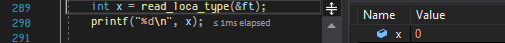the value is 0 meaning we have the short 16 bit sized version of the loca table

now to read the offset from loca is a matter of indexing the loca using the Glyph Index we get from get_glyph_index so we have this snippet.

 ``` 1 2 3 4 5 6 7 8 9 10``` ```u32 get_glyph_offset(font_directory *ft, u32 glyph_index) { u32 offset = 0; if(read_loca_type(ft)) { //32 bit offset = READ_BE32((u32*)ft->loca + glyph_index); } else { offset = READ_BE16((u16*)ft->loca + glyph_index)*2; } return offset; } ```

given that the output for this function isn't very exciting but just to check your code for the Glyph Index 622 which is the letter A the offset is 69700;

we will take a moment here to rewrite our get_glyph_index function to take a Codepoint and font_directory instead of taking the format4 directly.

 ``` 1 2 3 4 5 6 7 8 9 10 11 12 13 14 15 16 17 18 19 20 21 22 23``` ```int get_glyph_index(font_directory* ft, u16 code_point) { format4 *f = ft->f4; int index = -1; u16 *ptr = NULL; for(int i = 0; i < f->segCountX2/2; i++) { if(f->endCode[i] > code_point) {index = i; break;}; } if(index == -1) return 0; if(f->startCode[index] < code_point) { if(f->idRangeOffset[index] != 0) { ptr = f->idRangeOffset + index + f->idRangeOffset[index]/2; ptr += code_point - f->startCode[index]; if(*ptr == 0) return 0; return *ptr + f->idDelta[index]; } else { return code_point + f->idDelta[index]; } } return 0; } ```

we are done with the loca table now, we move onto the glyf table which is our last section

12. Where the wild glyfs are

This is the last section of our Part 1 and its extracting the Glyph data more commonly called Glyph Outline and as always we start with the structure,

 ```1 2 3 4 5 6 7``` ```typedef struct { u16 numberOfContours; i16 xMin; i16 yMin; i16 xMax; i16 yMax; }; ```

this isn't a complete struct, the data that follows depends on the numberOfContours field, if numberOfContours is bigger than zero it means its a simple Glyph if its less than zero it means its a componded Glyph meaning its made up of one or more Glyphs with a certain amount of transformations applied, we will have a gander at compound Glyphs in Part 1.5 (which might be after Part 2).

so for simple Glyphs we have the following structure:

 ``` 1 2 3 4 5 6 7 8 9 10 11 12 13``` ```typedef struct { u16 numberOfContours; i16 xMin; i16 yMin; i16 xMax; i16 yMax; u16 instructionLength; u8* instructions; u8* flags; i16* xCoordinates; i16* yCoordinates; u16* endPtsOfContours; } glyph_outline; ```

if you go ahead and check the reference we are using for this, you will see that the xCoordinates and yCoordinates could either be a u8 or i16 depending on the flags for making things easier we are just going to use the i16 because it will accommodate both sizes.

the numberOfContours tells us how many contours this shape has, it also gives us the size of endPtsOfContours, the endPtsOfContours array gives us the indicies of the end points of the contours i.e: endPtsOfContours gives us the index into xCoordinates and yCoordinates array where the first contour ends and endPtsOfContours gives us the index where the 2nd contour ends.
This means the last value in endPtsContour array will give us the number of points all the contours combined have thus we can allocate enough memory for all for all of them.

the intructionLength and instructions together gives us the instructions that are needed to do grid fitting (hinting) for this Glyph.
We won't be doing this as it requires us to code a complete virtual machine to run the instructions but its a fun project to do so maybe for another time.

the flags array gives us information about each of the points that the Glyph has, each flag is 8 bits and each bit has the following meanings:
• Bit 1: if set it means this point is on the Glyphs curve, otherwise the point is off curve.
• Bit 2: if set the corrosponding x coordiante is 1 byte otherwise its 2 bytes.
• Bit 3: if set the corrosponding y coordinate is 1 byte otherwise its 2 bytes.
• Bit 4: (repeat) if set the next byte specifies how many timet his flag repeats. this is a small way to compress the flags array.
• Bit 5, 6: these both relate to bit 1 and 2 respectivily and are better explained in a table.
• Bit 7, 8: those are reserved and set to zero in TrueType.

the bits (2, 5) and (3, 6) both have the same functionality but one is for the x coordinate the other is for y.
if we combine them then 2 bits give us 4 possibilities so here they are:

• a value of (0) means The current coordinate is 16 bit signed delta change.
• a value of (1) means The current coordinate is 16 bit, has the same value as the previous one.
• a value of (2) means the current coordinate is 8 bit, value is negative.
• a value of (3) means the current coordinate is 8 bit, value is positive.

we are going to use a union that has bitfields to help us make life easier when dealing with these.

 ``` 1 2 3 4 5 6 7 8 9 10 11 12 13 14 15 16 17``` ```typedef union { typedef struct { u8 on_curve: 1; u8 x_short: 1; u8 y_short: 1; u8 repeat: 1; u8 x_short_pos: 1; u8 y_short_pos: 1; u8 reserved1: 1; u8 reserved2: 1; }; u8 flag; } glyph_flag; ```

we will replace the type of the flags field in the glyph_outline struct to be of glyph_flag this will be easier for some coding.

this is all the information we need to read the Glyph outline correctly, we first read and uncompress the flags then we read the coordinates.

first the entire function:

 ``` 1 2 3 4 5 6 7 8 9 10 11 12 13 14 15 16 17 18 19 20 21 22 23 24 25 26 27 28 29 30 31 32 33 34 35 36 37 38 39 40 41 42 43 44 45 46 47 48 49 50 51 52 53 54 55 56 57 58 59 60 61 62 63 64 65 66 67 68 69 70 71 72 73 74``` ```glyph_outline get_glyph_outline(font_directory* ft, u32 glyph_index) { u32 offset = get_glyph_offset(ft, glyph_index); char* glyph_start = ft->glyf + offset; glyph_outline outline = {0}; outline.numberOfContours = READ_BE16_MOVE(glyph_start); outline.xMin = READ_BE16_MOVE(glyph_start); outline.yMin = READ_BE16_MOVE(glyph_start); outline.xMax = READ_BE16_MOVE(glyph_start); outline.yMax = READ_BE16_MOVE(glyph_start); outline.endPtsOfContours = (u16*) calloc(1, outline.numberOfContours*sizeof(u16)); for(int i = 0; i < outline.numberOfContours; ++i) { outline.endPtsOfContours[i] = READ_BE16_MOVE(glyph_start); } outline.instructionLength = READ_BE16_MOVE(glyph_start); outline.instructions = (u8*)calloc(1, outline.instructionLength); memcpy(outline.instructions, glyph_start, outline.instructionLength); P_MOVE(glyph_start, outline.instructionLength); int last_index = outline.endPtsOfContours[outline.numberOfContours-1]; outline.flags = (glyph_flag*) calloc(1, last_index + 1); for(int i = 0; i < (last_index + 1); ++i) { outline.flags[i].flag = *glyph_start; glyph_start++; if(outline.flags[i].repeat) { u8 repeat_count = *glyph_start; while(repeat_count-- > 0) { i++; outline.flags[i] = outline.flags[i-1]; } glyph_start++; } } outline.xCoordinates = (i16*) calloc(1, (last_index+1)*2); i16 prev_coordinate = 0; i16 current_coordinate = 0; for(int i = 0; i < (last_index+1); ++i) { int flag_combined = outline.flags[i].x_short << 1 | outline.flags[i].x_short_pos; switch(flag_combined) { case 0: { current_coordinate = READ_BE16_MOVE(glyph_start); } break; case 1: { current_coordinate = 0; }break; case 2: { current_coordinate = (*(u8*)glyph_start++)*-1; }break; case 3: { current_coordinate = (*(u8*)glyph_start++); } break; } outline.xCoordinates[i] = current_coordinate + prev_coordinate; prev_coordinate = outline.xCoordinates[i]; } outline.yCoordinates = (i16*) calloc(1, (last_index+1)*2); current_coordinate = 0; prev_coordinate = 0; for(int i = 0; i < (last_index+1); ++i) { int flag_combined = outline.flags[i].y_short << 1 | outline.flags[i].y_short_pos; switch(flag_combined) { case 0: { current_coordinate = READ_BE16_MOVE(glyph_start); } break; case 1: { current_coordinate = 0; }break; case 2: { current_coordinate = (*(u8*)glyph_start++)*-1; }break; case 3: { current_coordinate = (*(u8*)glyph_start++); } break; } outline.yCoordinates[i] = current_coordinate + prev_coordinate; prev_coordinate = outline.yCoordinates[i]; } return outline; } ```

one of our bigger functions so lets break it down:

 ``` 1 2 3 4 5 6 7 8 9 10 11 12 13 14 15 16 17 18 19``` ```u32 offset = get_glyph_offset(ft, glyph_index); char* glyph_start = ft->glyf + offset; glyph_outline outline = {0}; outline.numberOfContours = READ_BE16_MOVE(glyph_start); outline.xMin = READ_BE16_MOVE(glyph_start); outline.yMin = READ_BE16_MOVE(glyph_start); outline.xMax = READ_BE16_MOVE(glyph_start); outline.yMax = READ_BE16_MOVE(glyph_start); outline.endPtsOfContours = (u16*) calloc(1, outline.numberOfContours*sizeof(u16)); for(int i = 0; i < outline.numberOfContours; ++i) { outline.endPtsOfContours[i] = READ_BE16_MOVE(glyph_start); } outline.instructionLength = READ_BE16_MOVE(glyph_start); outline.instructions = (u8*)calloc(1, outline.instructionLength); memcpy(outline.instructions, glyph_start, outline.instructionLength); P_MOVE(glyph_start, outline.instructionLength); ```

this part is pretty straight forward, we get the offset of the Glyph and position glyph_start to be at the start of that Glyphs data. We start a new glyph_outline struct and read the easier fields. the instructions are just an arary of bytes so doing a memcpy is a lot faster than looping over memory.

now the part the reads the flags array

 ``` 1 2 3 4 5 6 7 8 9 10 11 12 13 14 15``` ```int last_index = outline.endPtsOfContours[outline.numberOfContours-1]; outline.flags = (glyph_flag*) calloc(1, last_index + 1); for(int i = 0; i < (last_index + 1); ++i) { outline.flags[i].flag = *glyph_start; glyph_start++; if(outline.flags[i].repeat) { u8 repeat_count = *glyph_start; while(repeat_count-- > 0) { i++; outline.flags[i] = outline.flags[i-1]; } glyph_start++; } } ```

we already know how big the flags array will be based on endPtsOfContours which gives us the last index of the last contour's point meaning the size of array is last_index + 1.

we read the flags one by one and everytime we encounter a repeat we read the next byte which gives us the amount we have to repeat.

now that we have the flags we can determine the x and y coordinates:

 ``` 1 2 3 4 5 6 7 8 9 10 11 12 13 14 15 16 17``` ```outline.xCoordinates = (i16*) calloc(1, (last_index+1)*2); i16 prev_coordinate = 0; i16 current_coordinate = 0; for(int i = 0; i < (last_index+1); ++i) { int flag_combined = outline.flags[i].x_short << 1 | outline.flags[i].x_short_pos; switch(flag_combined) { case 0: { current_coordinate = READ_BE16_MOVE(glyph_start); } break; case 1: { current_coordinate = 0; }break; case 2: { current_coordinate = (*glyph_start++)*-1; }break; case 3: { current_coordinate = (*glyph_start++); } break; } outline.xCoordinates[i] = current_coordinate + prev_coordinate; prev_coordinate = outline.xCoordinates[i]; } ```

this code is the same for both xCoordinates and yCoordinates, every coordinate point is relative to the previous one except the first which relative to 0 which means at each iteration the value for the current coordinate is the previous value summed with the data we just read. Under case 1 its indicated that the value of the current coordinate is the same as previous thus we don't have any data to read.

we repeat the same loop for the yCoordiantes and that is it.

now to print the outline.
 ``` 1 2 3 4 5 6 7 8 9 10 11 12 13``` ```void print_glyph_outline(glyph_outline *outline) { printf("#contours\t(xMin,yMin)\t(xMax,yMax)\tinst_length\n"); printf("%9d\t(%d,%d)\t\t(%d,%d)\t%d\n", outline->numberOfContours, outline->xMin, outline->yMin, outline->xMax, outline->yMax, outline->instructionLength); printf("#)\t( x , y )\n"); int last_index = outline->endPtsOfContours[outline->numberOfContours-1]; for(int i = 0; i <= last_index; ++i) { printf("%d)\t(%5d,%5d)\n", i, outline->xCoordinates[i], outline->yCoordinates[i]); } } ```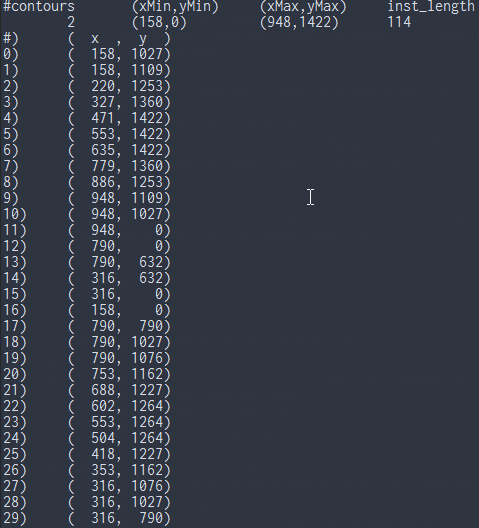and that is all she wrote. (for now)

13. Is it Over?

There is still Part 2 coming which will be how to rasierize this outline, it will include initializing OpengGL Core profile on Windows nativily without any libraries, loading the OpenGL functions we need, Scanline Raserization of our outline, some basic AA.

as a wrap-up here I'm going to include the things we haven't covered.

1. we didn't touch the head, hhea and hmtx and the other tables that relate to positioning of Glyphs I will try to include those in a seperate post so that this doesn't really become too big of a post.
2. We skipped reading any platformID, platformSpecificID pair other than (0, 3)
3. We skipped reading any cmap table format other than format 4
4. We skipped reading Comounded Glyph data and just focused on simple Glyphs
5. We skipped doing anything we the instructions we read for a Glyph

I will try to come back to these points at a later date, possibily in Part 1.5 so that this post can be more complete
----
 in order to convert a Fixed number into our normal float types, we first need to figure what our fraction is which is the lower 16 bits divided by (2^16) we then simply add this to our whole part and we get the number in float. In OpenType this version is actually divided back into 2 16 bit fields, one called Major version and the other Minor version.
 every font is designed on a 2D grid whose coordinates are in FUnits the size of this grid is 1 em, and how many FUnits are in this 1 em is defined by the unitsPerEm field of the head table, the higher this count the more precision font designers have.

Here is all the code combined:

 ``` 1 2 3 4 5 6 7 8 9 10 11 12 13 14 15 16 17 18 19 20 21 22 23 24 25 26 27 28 29 30 31 32 33 34 35 36 37 38 39 40 41 42 43 44 45 46 47 48 49 50 51 52 53 54 55 56 57 58 59 60 61 62 63 64 65 66 67 68 69 70 71 72 73 74 75 76 77 78 79 80 81 82 83 84 85 86 87 88 89 90 91 92 93 94 95 96 97 98 99 100 101 102 103 104 105 106 107 108 109 110 111 112 113 114 115 116 117 118 119 120 121 122 123 124 125 126 127 128 129 130 131 132 133 134 135 136 137 138 139 140 141 142 143 144 145 146 147 148 149 150 151 152 153 154 155 156 157 158 159 160 161 162 163 164 165 166 167 168 169 170 171 172 173 174 175 176 177 178 179 180 181 182 183 184 185 186 187 188 189 190 191 192 193 194 195 196 197 198 199 200 201 202 203 204 205 206 207 208 209 210 211 212 213 214 215 216 217 218 219 220 221 222 223 224 225 226 227 228 229 230 231 232 233 234 235 236 237 238 239 240 241 242 243 244 245 246 247 248 249 250 251 252 253 254 255 256 257 258 259 260 261 262 263 264 265 266 267 268 269 270 271 272 273 274 275 276 277 278 279 280 281 282 283 284 285 286 287 288 289 290 291 292 293 294 295 296 297 298 299 300 301 302 303 304 305 306 307 308 309 310 311 312 313 314 315 316 317 318 319 320 321 322 323 324 325 326 327 328 329 330 331 332 333 334 335 336 337 338 339 340 341 342 343 344 345 346 347 348 349 350 351 352 353 354 355 356 357 358 359 360 361 362 363 364 365 366 367 368 369 370 371 372 373 374 375 376 377 378 379 380 381 382 383 384 385 386 387 388 389 390 391 392 393 394 395 396 397 398 399 400 401 402 403 404 405 406 407 408 409 410 411 412 413 414 415 416 417 418 419 420 421 422 423 424``` ```#include #define READ_BE16(mem) ((((u8*)(mem)) << 8) | (((u8*)(mem)))) #define READ_BE32(mem) ((((u8*)(mem)) << 24) | (((u8*)(mem)) << 16) | (((u8*)(mem)) << 8) | (((u8*)(mem)))) #define P_MOVE(mem, a) ((mem) += (a)) #define READ_BE16_MOVE(mem) (READ_BE16((mem))); (P_MOVE((mem), 2)) #define READ_BE32_MOVE(mem) (READ_BE32((mem))); (P_MOVE((mem), 4)) #define FONT_TAG(a, b, c, d) (a<<24|b<<16|c<<8|d) #define GLYF_TAG FONT_TAG('g', 'l', 'y', 'f') #define LOCA_TAG FONT_TAG('l', 'o', 'c', 'a') #define HEAD_TAG FONT_TAG('h', 'e', 'a', 'd') #define CMAP_TAG FONT_TAG('c', 'm', 'a', 'p') char* read_file(char *file_name, int* file_size) { if(strlen(file_name) > 0) { FILE* file = fopen(file_name, "rb"); if(file) { fseek(file, 0, SEEK_END); int size = ftell(file); fseek(file, 0, SEEK_SET); if(file_size) { *file_size = size; } char *file_content = (char*)malloc(size+1); int read_amount = fread(file_content, size, 1, file); file_content[size] = '\0'; if(read_amount) { fclose(file); return file_content; } free(file_content); fclose(file); return NULL; } } return NULL; } typedef unsigned char u8; typedef char i8; typedef unsigned short u16; typedef short i16; typedef unsigned int u32; typedef int i32; typedef struct { u32 scaler_type; u16 numTables; u16 searchRange; u16 entrySelector; u16 rangeShift; } offset_subtable; typedef struct { u16 platformID; u16 platformSpecificID; u32 offset; } cmap_encoding_subtable; typedef struct { u16 version; u16 numberSubtables; cmap_encoding_subtable* subtables; } cmap; typedef struct { u16 format; u16 length; u16 language; u16 segCountX2; u16 searchRange; u16 entrySelector; u16 rangeShift; u16 reservedPad; u16 *endCode; u16 *startCode; u16 *idDelta; u16 *idRangeOffset; u16 *glyphIdArray; } format4; typedef struct { union { char tag_c; u32 tag; }; u32 checkSum; u32 offset; u32 length; } table_directory; typedef struct { offset_subtable off_sub; table_directory* tbl_dir; format4* f4; cmap* cmap; char* glyf; char* loca; char* head; } font_directory; typedef union { typedef struct { u8 on_curve: 1; u8 x_short: 1; u8 y_short: 1; u8 repeat: 1; u8 x_short_pos: 1; u8 y_short_pos: 1; u8 reserved1: 1; u8 reserved2: 1; }; u8 flag; } glyph_flag; typedef struct { u16 numberOfContours; i16 xMin; i16 yMin; i16 xMax; i16 yMax; u16 instructionLength; u8* instructions; glyph_flag* flags; i16* xCoordinates; i16* yCoordinates; u16* endPtsOfContours; } glyph_outline; void read_offset_subtable(char** mem, offset_subtable* off_sub) { char* m = *mem; off_sub->scaler_type = READ_BE32_MOVE(m); off_sub->numTables = READ_BE16_MOVE(m); off_sub->searchRange = READ_BE16_MOVE(m); off_sub->entrySelector = READ_BE16_MOVE(m); off_sub->rangeShift = READ_BE16_MOVE(m); *mem = m; } void read_cmap(char* mem, cmap* c) { char *m = mem; c->version = READ_BE16_MOVE(m); c->numberSubtables = READ_BE16_MOVE(m); c->subtables = (cmap_encoding_subtable*) calloc(1, sizeof(cmap_encoding_subtable)*c->numberSubtables); for(int i = 0; i < c->numberSubtables; ++i) { cmap_encoding_subtable* est = c->subtables + i; est->platformID = READ_BE16_MOVE(m); est->platformSpecificID = READ_BE16_MOVE(m); est->offset = READ_BE32_MOVE(m); } } void print_cmap(cmap* c) { printf("#)\tpId\tpsID\toffset\ttype\n"); for(int i = 0; i < c->numberSubtables; ++i) { cmap_encoding_subtable* cet = c->subtables + i; printf("%d)\t%d\t%d\t%d\t", i+1, cet->platformID, cet->platformSpecificID, cet->offset); switch(cet->platformID) { case 0: printf("Unicode"); break; case 1: printf("Mac"); break; case 2: printf("Not Supported"); break; case 3: printf("Microsoft"); break; } printf("\n"); } } void read_format4(char* mem, format4** format) { char* m = mem; u16 length = READ_BE16(m + 2); format4* f = NULL; f = (format4*) calloc(1, length + sizeof(u16*)*5); f->format = READ_BE16_MOVE(m); f->length = READ_BE16_MOVE(m); f->language = READ_BE16_MOVE(m); f->segCountX2 = READ_BE16_MOVE(m); f->searchRange = READ_BE16_MOVE(m); f->entrySelector = READ_BE16_MOVE(m); f->rangeShift = READ_BE16_MOVE(m); f->endCode = (u16*) ((u8*)f + sizeof(format4)); f->startCode = f->endCode + f->segCountX2/2; f->idDelta = f->startCode + f->segCountX2/2; f->idRangeOffset = f->idDelta + f->segCountX2/2; f->glyphIdArray = f->idRangeOffset + f->segCountX2/2; char* start_code_start = m + f->segCountX2 + 2; char* id_delta_start = m + f->segCountX2*2 + 2; char* id_range_start = m + f->segCountX2*3 + 2; for(int i = 0; i < f->segCountX2/2; ++i) { f->endCode[i] = READ_BE16(m + i*2); f->startCode[i] = READ_BE16(start_code_start + i*2); f->idDelta[i] = READ_BE16(id_delta_start + i*2); f->idRangeOffset[i] = READ_BE16(id_range_start + i*2); } P_MOVE(m, f->segCountX2*4 + 2); int remaining_bytes = f->length - (m - mem); for(int i = 0; i < remaining_bytes/2; ++i) { f->glyphIdArray[i] = READ_BE16_MOVE(m); } *format = f; } void print_format4(format4 *f4) { printf("Format: %d, Length: %d, Language: %d, Segment Count: %d\n", f4->format, f4->length, f4->language, f4->segCountX2/2); printf("Search Params: (searchRange: %d, entrySelector: %d, rangeShift: %d)\n", f4->searchRange, f4->entrySelector, f4->rangeShift); printf("Segment Ranges:\tstartCode\tendCode\tidDelta\tidRangeOffset\n"); for(int i = 0; i < f4->segCountX2/2; ++i) { printf("--------------:\t% 9d\t% 7d\t% 7d\t% 12d\n", f4->startCode[i], f4->endCode[i], f4->idDelta[i], f4->idRangeOffset[i]); } } void read_table_directory(char* file_start, char** mem, font_directory* ft) { char* m = *mem; ft->tbl_dir = (table_directory*)calloc(1, sizeof(table_directory)*ft->off_sub.numTables); for(int i = 0; i < ft->off_sub.numTables; ++i) { table_directory* t = ft->tbl_dir + i; t->tag = READ_BE32_MOVE(m); t->checkSum = READ_BE32_MOVE(m); t->offset = READ_BE32_MOVE(m); t->length = READ_BE32_MOVE(m); switch(t->tag) { case GLYF_TAG: ft->glyf = t->offset + file_start; break; case LOCA_TAG: ft->loca = t->offset + file_start; break; case HEAD_TAG: ft->head = t->offset + file_start; break; case CMAP_TAG: { ft->cmap = (cmap*) calloc(1, sizeof(cmap)); read_cmap(file_start + t->offset, ft->cmap); read_format4(file_start + t->offset + ft->cmap->subtables.offset, &ft->f4); } break; } } *mem = m; } void print_table_directory(table_directory* tbl_dir, int tbl_size) { printf("#)\ttag\tlen\toffset\n"); for(int i = 0; i < tbl_size; ++i) { table_directory* t = tbl_dir + i; printf("%d)\t%c%c%c%c\t%d\t%d\n", i+1, t->tag_c, t->tag_c, t->tag_c, t->tag_c, t->length, t->offset); } } void read_font_directory(char* file_start, char** mem, font_directory* ft) { read_offset_subtable(mem, &ft->off_sub); read_table_directory(file_start, mem, ft); } int get_glyph_index(font_directory* ft, u16 code_point) { format4 *f = ft->f4; int index = -1; u16 *ptr = NULL; for(int i = 0; i < f->segCountX2/2; i++) { if(f->endCode[i] > code_point) {index = i; break;}; } if(index == -1) return 0; if(f->startCode[index] < code_point) { if(f->idRangeOffset[index] != 0) { ptr = f->idRangeOffset + index + f->idRangeOffset[index]/2; ptr += code_point - f->startCode[index]; if(*ptr == 0) return 0; return *ptr + f->idDelta[index]; } else { return code_point + f->idDelta[index]; } } return 0; } int read_loca_type(font_directory* ft) { return READ_BE16(ft->head + 50); } u32 get_glyph_offset(font_directory *ft, u32 glyph_index) { u32 offset = 0; if(read_loca_type(ft)) { //32 bit offset = READ_BE32((u32*)ft->loca + glyph_index); } else { offset = READ_BE16((u16*)ft->loca + glyph_index)*2; } return offset; } glyph_outline get_glyph_outline(font_directory* ft, u32 glyph_index) { u32 offset = get_glyph_offset(ft, glyph_index); unsigned char* glyph_start = ft->glyf + offset; glyph_outline outline = {0}; outline.numberOfContours = READ_BE16_MOVE(glyph_start); outline.xMin = READ_BE16_MOVE(glyph_start); outline.yMin = READ_BE16_MOVE(glyph_start); outline.xMax = READ_BE16_MOVE(glyph_start); outline.yMax = READ_BE16_MOVE(glyph_start); outline.endPtsOfContours = (u16*) calloc(1, outline.numberOfContours*sizeof(u16)); for(int i = 0; i < outline.numberOfContours; ++i) { outline.endPtsOfContours[i] = READ_BE16_MOVE(glyph_start); } outline.instructionLength = READ_BE16_MOVE(glyph_start); outline.instructions = (u8*)calloc(1, outline.instructionLength); memcpy(outline.instructions, glyph_start, outline.instructionLength); P_MOVE(glyph_start, outline.instructionLength); int last_index = outline.endPtsOfContours[outline.numberOfContours-1]; outline.flags = (glyph_flag*) calloc(1, last_index + 1); for(int i = 0; i < (last_index + 1); ++i) { outline.flags[i].flag = *glyph_start; glyph_start++; if(outline.flags[i].repeat) { u8 repeat_count = *glyph_start; while(repeat_count-- > 0) { i++; outline.flags[i] = outline.flags[i-1]; } glyph_start++; } } outline.xCoordinates = (i16*) calloc(1, (last_index+1)*2); i16 prev_coordinate = 0; i16 current_coordinate = 0; for(int i = 0; i < (last_index+1); ++i) { int flag_combined = outline.flags[i].x_short << 1 | outline.flags[i].x_short_pos; switch(flag_combined) { case 0: { current_coordinate = READ_BE16_MOVE(glyph_start); } break; case 1: { current_coordinate = 0; }break; case 2: { current_coordinate = (*glyph_start++)*-1; }break; case 3: { current_coordinate = (*glyph_start++); } break; } outline.xCoordinates[i] = current_coordinate + prev_coordinate; prev_coordinate = outline.xCoordinates[i]; } outline.yCoordinates = (i16*) calloc(1, (last_index+1)*2); current_coordinate = 0; prev_coordinate = 0; for(int i = 0; i < (last_index+1); ++i) { int flag_combined = outline.flags[i].y_short << 1 | outline.flags[i].y_short_pos; switch(flag_combined) { case 0: { current_coordinate = READ_BE16_MOVE(glyph_start); } break; case 1: { current_coordinate = 0; }break; case 2: { current_coordinate = (*glyph_start++)*-1; }break; case 3: { current_coordinate = (*glyph_start++); } break; } outline.yCoordinates[i] = current_coordinate + prev_coordinate; prev_coordinate = outline.yCoordinates[i]; } return outline; } void print_glyph_outline(glyph_outline *outline) { printf("#contours\t(xMin,yMin)\t(xMax,yMax)\tinst_length\n"); printf("%9d\t(%d,%d)\t\t(%d,%d)\t%d\n", outline->numberOfContours, outline->xMin, outline->yMin, outline->xMax, outline->yMax, outline->instructionLength); printf("#)\t( x , y )\n"); int last_index = outline->endPtsOfContours[outline->numberOfContours-1]; for(int i = 0; i <= last_index; ++i) { printf("%d)\t(%5d,%5d)\n", i, outline->xCoordinates[i], outline->yCoordinates[i]); } } int main(int argc, char** argv) { int file_size = 0; char* file = read_file("envy.ttf", &file_size); char* mem_ptr = file; font_directory ft = {0}; read_font_directory(file, &mem_ptr, &ft); glyph_outline A = get_glyph_outline(&ft, get_glyph_index(&ft, 'A')); print_glyph_outline(&A); return 0; } ```

XX. Why did this take so long?
The main reason is nothing other than self-doubt, not considereing myself good enough and sprinkle of depression on top that coupled with having to change jobs and move a city over from home really all combined itself into one giant mess. I have always had a lot of self-hate for myself most relating to childhood up bringing and what have you however all of that got better for me, a few months ago I couldn't sit down on my computer and write 3 lines of code without having intrusive thoughts about my own skill so I just couldn't write anything but with some proper self reflection and meditation I was able to (as cliche as it sounds) accept my own skill level, now I couldn't be happier I have spent this week and the last one doing 12 hour coding per day and its a blessing. Out of everything the one thing that helped the most were videos by (Dr. Alok Kanojia) over at HealthyGamer, his work helped me a lot.
so why am I writing this part? simply because it could be of use to others, others who might be going through a similar thing and maybe this could help them in some form.

memes & pepe will be the downfall of mankind.
1 posts
Implementing a Font Reader and Rasterizer from Scratch, Part 1: TTF Font Reader.
8 months, 2 weeks ago
Hey, thanks for sharing this.

Maybe add an intro paragraph like:

True Type Font (TTF) files are the most common format for storing and sharing fonts across all modern computer systems. In this tutorial we will be exploring this format to read font data from .ttf files and rasterize them for use in games or other applications.

Also, the first link is wrong, it should be:
 `1` ```https://developer.apple.com/fonts/TrueType-Reference-Manual/RM06/Chap6.html ```

If you don't mind, after I read through the full tutorial I could edit it to be a bit clearer and to clean up some typos? Some parts seem a bit hard to follow. Thanks again.
Simon Anciaux
943 posts
Implementing a Font Reader and Rasterizer from Scratch, Part 1: TTF Font Reader.
8 months, 2 weeks ago Edited by Simon Anciaux on May 13, 2020, 12:57 p.m.
Thanks for taking the time to write this.

I agree with KevinM, some places could use some proofreading.
S
15 posts

Implementing a Font Reader and Rasterizer from Scratch, Part 1: TTF Font Reader.
8 months, 2 weeks ago
You are welcome, I hope its of use.
Nice intro, will wait until more corrections come up and add them all together.

Weird how the word "True" was just missing from the link, I blame one vim plugin I used that deals with markdown files, the post is in MD format on my PC. I will fix it.

I wouldn't mind any corrections but just let me know what you are changing before hand.

memes & pepe will be the downfall of mankind.
S
15 posts

Implementing a Font Reader and Rasterizer from Scratch, Part 1: TTF Font Reader.
8 months, 2 weeks ago
By all means I'm open to any and all corrections, English isn't my first language.

memes & pepe will be the downfall of mankind.
Alexey
9 posts
Implementing a Font Reader and Rasterizer from Scratch, Part 1: TTF Font Reader.
6 months ago Edited by Alexey on July 29, 2020, 6:08 p.m. Reason: i'm dumb
Thanks for the great reference! I've been fiddling with TTF's for the last few days and your code has been very helpful.

A few small corrections:

1. When computing glyph offsets, idDelta arithmetic should be modulo 0xFFFF, as per spec:

https://developer.apple.com/fonts...erence-Manual/RM06/Chap6cmap.html
NOTE: All idDelta arithmetic is modulo 65536.

It is and important note, and your implementation doesn't work (I guess because of integer promotion), you write:
 ```1 2 3``` ```return *ptr + f->idDelta[index]; // and return code_point + f->idDelta[index]; ```

Which should be:
 ```1 2 3``` ```return (s16) (*ptr + f->idDelta[index]); // and return (s16) (code_point + f->idDelta[index]); ```

2. Your final listing misses just a few things to be runnable: includes of string.h and stdlib.h, and also there's a typo here (inner typedef is not needed):
 ```1 2 3 4 5 6 7``` ```typedef union { typedef struct { u8 on_curve: 1; ... }; u8 flag; } glyph_flag; ```

Other than that everything seems alright. Thank you again for saving me probably a dozen hours! Have a nice day
S
15 posts

Implementing a Font Reader and Rasterizer from Scratch, Part 1: TTF Font Reader.
5 months, 3 weeks ago
aolo2
Thanks for the great reference! I've been fiddling with TTF's for the last few days and your code has been very helpful.

A few small corrections:

1. When computing glyph offsets, idDelta arithmetic should be modulo 0xFFFF, as per spec:

https://developer.apple.com/fonts...erence-Manual/RM06/Chap6cmap.html
NOTE: All idDelta arithmetic is modulo 65536.

It is and important note, and your implementation doesn't work (I guess because of integer promotion), you write:
 ```1 2 3``` ```return *ptr + f->idDelta[index]; // and return code_point + f->idDelta[index]; ```

Which should be:
 ```1 2 3``` ```return (s16) (*ptr + f->idDelta[index]); // and return (s16) (code_point + f->idDelta[index]); ```

2. Your final listing misses just a few things to be runnable: includes of string.h and stdlib.h, and also there's a typo here (inner typedef is not needed):
 ```1 2 3 4 5 6 7``` ```typedef union { typedef struct { u8 on_curve: 1; ... }; u8 flag; } glyph_flag; ```

Other than that everything seems alright. Thank you again for saving me probably a dozen hours! Have a nice day

Hey thanks for the pointers:
1- the arithmetic is indeed module 65536 however I'm using uint 16 which when it overflows it actually does a module of 65536, the C standard states this, in my own implementation I actually have the module in there however when I rewrote this a 2nd time I was like "oh overflows work like module" hence why I didn't include it here but I should have mentioned it, thanks.

2- I could have sworn the code compiled correctly when I wrote it, I always double check the code by copy pasting what is in the forum and trying to compile it, but yes you are right I had to include both stdlib and string for it to compile correctly this time. Thank you for pointing this out.

memes & pepe will be the downfall of mankind.
Mārtiņš Možeiko
2162 posts / 1 project
Implementing a Font Reader and Rasterizer from Scratch, Part 1: TTF Font Reader.
5 months, 3 weeks ago Edited by Mārtiņš Možeiko on Aug. 7, 2020, 8:39 p.m.
Don't forget that in C when you add two u16's the result has int type. So there is no "overflow". Only if you assign result back to u16 it will do %65536 equivalent operation.
Alexey
9 posts
Implementing a Font Reader and Rasterizer from Scratch, Part 1: TTF Font Reader.
5 months, 3 weeks ago Edited by Alexey on Aug. 10, 2020, 7:32 a.m. Reason: being more verbose
Exactly, the return type is int, so the mod 65536 never happens. The arguments of the addition are implicitly promoted to int - that's what I referred to as integer promotion.
1 posts
Implementing a Font Reader and Rasterizer from Scratch, Part 1: TTF Font Reader.
4 months, 2 weeks ago
I made an account just to say thank you for doing this. I've wanted to understand font files for a long time since the library options for reading font files seem very limited but I had trouble understanding the TTF spec. I've heard that font files are a nightmare to parse but I didn't quite understand just how big of a disaster they are until now.

It seems to me like a great example of some ancient construct from a time before we knew what we were doing and needs to be replaced with something simpler. My job involves lots of fonts and I've seen many fonts that behave in strange ways in certain programs or just downright break and then work fine on another program, and I think it's probably because the file format is such a mess. Even just to get the damn font name you need to search across tables inside tables inside tables for various platforms and text encoding formats, what if some hardcode apple fan will only fill the Mac specific tables and puts some placeholders in the others? You'd have to do some really esoteric tricks to fully support all fonts.

Anyway I drew lines across the glyf X/Y coordinates and what came out does indeed look like the outlines for 'A' from the given font (lack of curves notwithstanding).